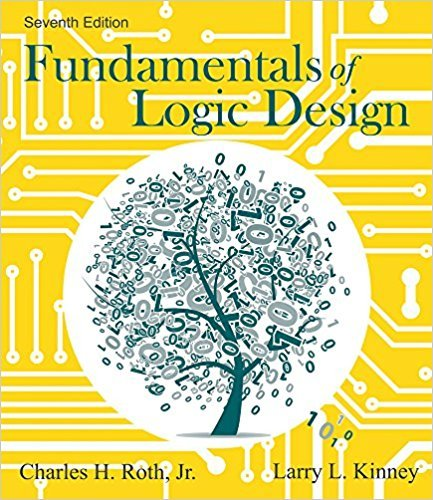×

×

# Solutions for Chapter 1: Introduction Number Systems and Conversion## Full solutions for Fundamentals of Logic Design | 7th Edition

ISBN: 9781133628477Solutions for Chapter 1: Introduction Number Systems and Conversion

Solutions for Chapter 1
4 5 0 369 Reviews
22
3
##### ISBN: 9781133628477

This expansive textbook survival guide covers the following chapters and their solutions. This textbook survival guide was created for the textbook: Fundamentals of Logic Design, edition: 7. Chapter 1: Introduction Number Systems and Conversion includes 46 full step-by-step solutions. Since 46 problems in chapter 1: Introduction Number Systems and Conversion have been answered, more than 63689 students have viewed full step-by-step solutions from this chapter. Fundamentals of Logic Design was written by and is associated to the ISBN: 9781133628477.

Key Engineering and Tech Terms and definitions covered in this textbook